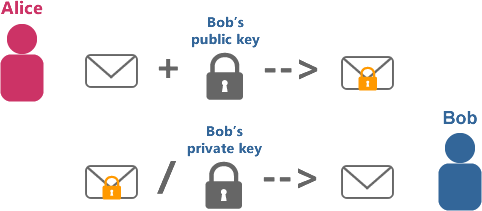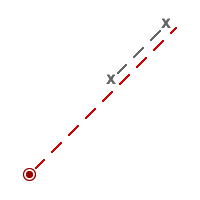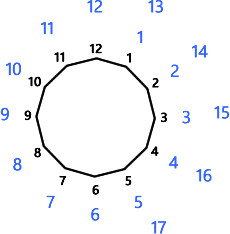# The beauty of asymmetric encryption - RSA crash course for developers

Published

With the rapid growth of the internet and the vast business which is handled over the web it is not surprising that security has become an inevitable topic for any software developer these days.

Unfortunately security and in particular cryptography is a complex science on its own. It is very difficult to get it right and extremely easy to get it wrong.

As a developer I personally find the topic very interesting and challenging and therefore strive to understand the concepts of cryptography more than just the bare minimum.

With this in mind I would like to break down one of the most important cryptographic algorithms which is used in modern web development nowadays: Asymmetric encryption with RSA.

## History

While modern cryptology has a long history dating back to AD 800, asymmetric encryption is only a very recent discovery starting in the mid-70s.

Previously every symmetric encryption algorithm had the fundamental problem of the secret key distribution. Two parties had to find a way to share a secret key via an insecure channel before they could successfully exchange private messages.

Only in 1977 (a year after Whitfield Diffie and Martin Hellman introduced the Diffie-Hellman key exchange algorithm) three scientists, Ron Rivest, Adi Shamir and Leonard Adleman, succeeded in inventing the first publicly known asymmetric encryption algorithm named RSA. Back then GCHQ, a UK intelligence agency has independently developed the first public key algorithm in 1973 and 1974, but this was kept secret until the mid 80s.

Today RSA is the de-facto standard asymmetric encryption algorithm and is used in many areas such as TLS/SSL, SSH, digital signatures and PGP.

## Basics

Before cracking down the RSA algorithm I would like to scratch on some basics, which are essential to understand the nature of RSA.

### Public-key encryption

Public-key encryption, as opposed to secret-key encryption, consists of a pair of keys - the public key which is used to encrypt a message and the private key, which is subsequently used to decrypt the cipher message.

Each private key has only one matching public key. A message encrypted with the public key can only be decrypted with the related private key.

#### Alice, Bob and Eve

Alice and Bob want to communicate privately and Eve wants to eavesdrop. Both, Alice and Bob have their individual public and private key pair.

Alice uses Bob's public key to encrypt a private message before sending it to Bob. Bob can use his private key to decrypt the message. Now Bob can use Alice's public key to reply to Alice without Eve being able to understand any of the transmitted data. Finally Alice decrypts Bob's message with her own private key.The public key is available to everyone, while the private key is only known to the key holder. There is never the requirement to share a secret key via an insecure channel.

#### Integrity and Authenticity

Now theoretically Eve could equally use Alice's or Bob's public key and send them a private message. It wouldn't help her directly to read anyone's encrypted message, but she could trick either one of them to believe her message was from Bob or Alice and provoke them to make a mistake.

Eve could also intercept and tamper with the encrypted message, so that after decryption it will read something different.

Just by encrypting a message neither Bob or Alice can ever be sure that the message hasn't been modified (integrity) by Eve nor that the message wasn't sent from Eve in the first place (authenticity).

Luckily RSA offers a solution to this problem. Similar to encrypting a message, the algorithm can also be used for signing it. Bob can use his private key to compute a signature from his message. The signature would be unique to the message and no other message would compute the same signature.

Alice can then use Bob's public key to verify if the message and the attached signature match. Because she uses Bob's public key she can be sure that the message came from Bob. Only Bob can create a correct signature with his private key. Additionally Alice will easily know if the message has been modified or not. If Eve changed the message or the attached signature, then Alice will not be able to verify the signature with Bob's public key and therefore know that someone has tampered with the data.

By providing an additional signature Alice and Bob can trust each others messages.

#### Encrypting and signing

Encrypting or signing are not exclusive. For example Alice can first encrypt her message and then use the resulting cipher as the base for computing a valid signature. Afterwards Bob has to first verify the signature based on the cipher and when that goes well he can proceed to decrypt the message. Now both have established a trusted and secure way of communicating privately.

### One-way functions

The concept of encrypting a message with one key and not being able to decrypt with the same key is based on one-way functions. As the name suggests the characteristic of a one-way function is that it is not reversible other than with a trial and error approach.

This can be achieved if there is an infinite amount of values which lead to the same result, if there is some information lost as part of the algorithm or if the time to decrypt takes immensely longer than to encrypt.

#### A simple example of a one-way function

Let's say the initial value is 264. The one-way function reads as following:

You start from the centre of a map. Now take your value and divide it by it's last digit. The result is a new value x. Now draw a line x centimetres north east and mark a new point on the map. Next take your original value and subtract it by x. The result is y. Draw another line, starting from the last point, y centimetres south west. The final point is the end result.In this example we would divide 264 by 4 and retrieve 66 for x. Additionally we subtract 66 from 264 and retrieve y = 198. We draw both lines and determine the final point on the map, which represents the end result of the one-way function.

Now just from knowing the final point on the map and the definition of the function it is not possible to easily deduce the original value.

### Modular arithmetic

Modular arithmetic is full of one-way functions. It is also known as clock arithmetic, because it can be illustrated by a finite amount of numbers arranged in a loop, like on a clock:The dark circle represents the clock. The blue numbers represent the value 17. If you arrange all numbers from 1 to 17 clockwise in a loop, then the end value results in 5. In other words 17 mod 12 equals 5.

The short-cut and common way of calculating the modulus is by dividing the original value by x. The reminder equals the modulus.

The modulus operation is a great one-way function, because it is fairly simple and has an infinite amount of possible values giving the same result.

### Prime numbers

Prime numbers are the last important building block of the RSA algorithm.

A prime number (or a prime) is a natural number greater than 1 that has no positive divisors other than 1 and itself.

Example of prime numbers: 2, 3, 5, 7, 11, 13, 17, 19, 23, 29, ...

What's important to know is that there is an infinite amount of prime numbers and there is no effective formula to calculate a prime number or to validate a given number to be prime or not.

### Prime factorisation

Factorisation is the process of decomposing a positive integer into a product of smaller numbers.

For example the number 759 can be decomposed into 3 and 253.
In other words 759 = 3 * 253.

If one continues the process you will eventually end up with all numbers being prime numbers (= prime factorisation). In our case 253 can be further broken down into 11 and 23.

Finally you can say 759 = 3 * 11 * 23.

Primes become rarer as we progress through the integers and there is a race in finding the next highest prime number in history.

## The RSA algorithm

As genius as the algorithm is, it is fairly short and can be demonstrated with a relatively small example.

### Generating the public and private key

First Alice needs to generate her own public and private key pair. For this Alice picks two prime numbers p = 17 and q = 19.

She multiplies them together to retrieve the number n = 323.

Next she picks another prime e = 7.

Lastly she needs to calculate the value d. This is done by working out the following equation:
e * d mod ((p - 1) * (q - 1)) = 1
7 * d mod 288 = 1
d = 247

#### Private key

The private key is d = 247.

p and q are kept secret as well. d can only be calculated if p and q are known.

#### Public key

The public key is formed by n and e:
n = 323
e = 7

Note that n is the product of two prime numbers. It can only be decomposed into p and q. If p and q are large enough then it is de-facto impossible.

### Encrypting a message

The public key is used to encrypt a message. Let's say Bob wants to encrypt the message "I love Alice". Internally all data is represented in binary format and binary numbers can be converted into decimals.

In C# you can quickly convert it using this snippet:

``````var message = "I love Alice";
var binary = Encoding.ASCII.GetBytes(message);
var decimals = binary.Select(b => Convert.ToInt32(b)).ToArray();``````

"I love Alice" is represented by this number sequence:
73, 32, 108, 111, 118, 101, 32, 65, 108, 105, 99, 101.

To keep this example short I will only encrypt the first letter of the message, which is represented by the number 73.

The formula to encrypt m = 73 is:
c = me mod n
c = 737 mod 323
The cipher c = 112.

### Decrypting the cipher

Alice receives the cipher 112 and can decrypt it using her private key d and the formula:
m = cd mod n
m = 112247 mod 323
The first number of the original message is m = 73.

RSA is a brilliant one-way function which allows someone to reverse it only if the private key d is known.

## Real world application

RSA is considerably slow due to the calculation with large numbers. In particular the decryption where d is used in the exponent is slow. There are ways to speed it up by remembering p and q, but it is still slow in comparison to symmetric encryption algorithms.

A common practise is to use RSA only for the encryption of a secret key, which then is used in a symmetric encryption algorithm. Typically the message to encrypt is a lot longer than the secret key itself, therefore this is a very effective method to benefit from the security of an asymmetric- and the speed of a symmetric encryption algorithm.

### Key length

With the fast development of computer chips the recommended key length for RSA changes over time.

RSA claims that 1024-bit keys are likely to become crackable some time between 2006 and 2010 and that 2048-bit keys are sufficient until 2030. An RSA key length of 3072 bits should be used if security is required beyond 2030.

If you use RSA in your application, then you should periodically (every few years) recycle your keys and generate a new pair to meet current length recommendations and stay secure.

## Future

The entire security of RSA is built on the fact that it is impractical to determine p and q by looking at n. If at some point in the future a mathematician finds a way to rapidly factor n, then RSA would become of no use.

Another interesting way of cracking most of today's crypto systems could be with the help of quantum computing, which as of now still remains a very theoretical topic.